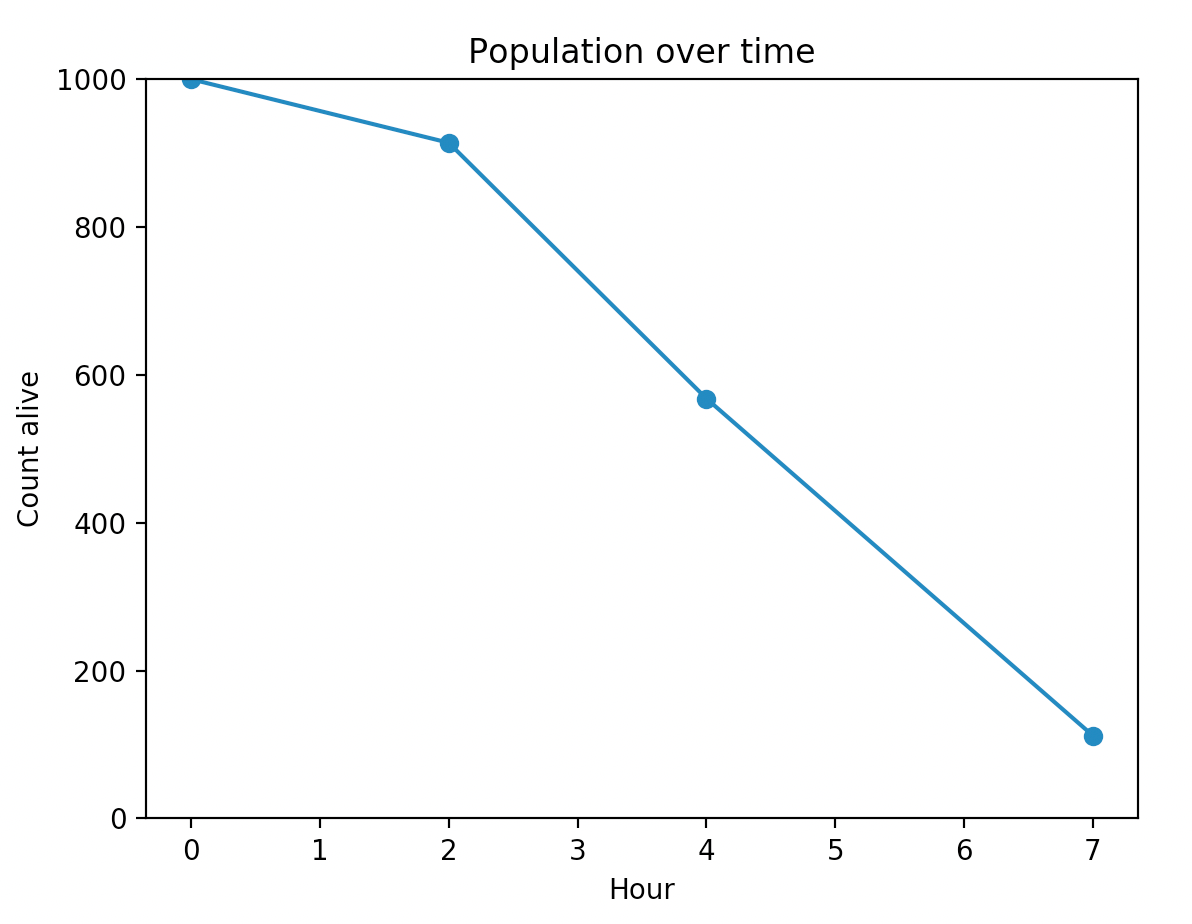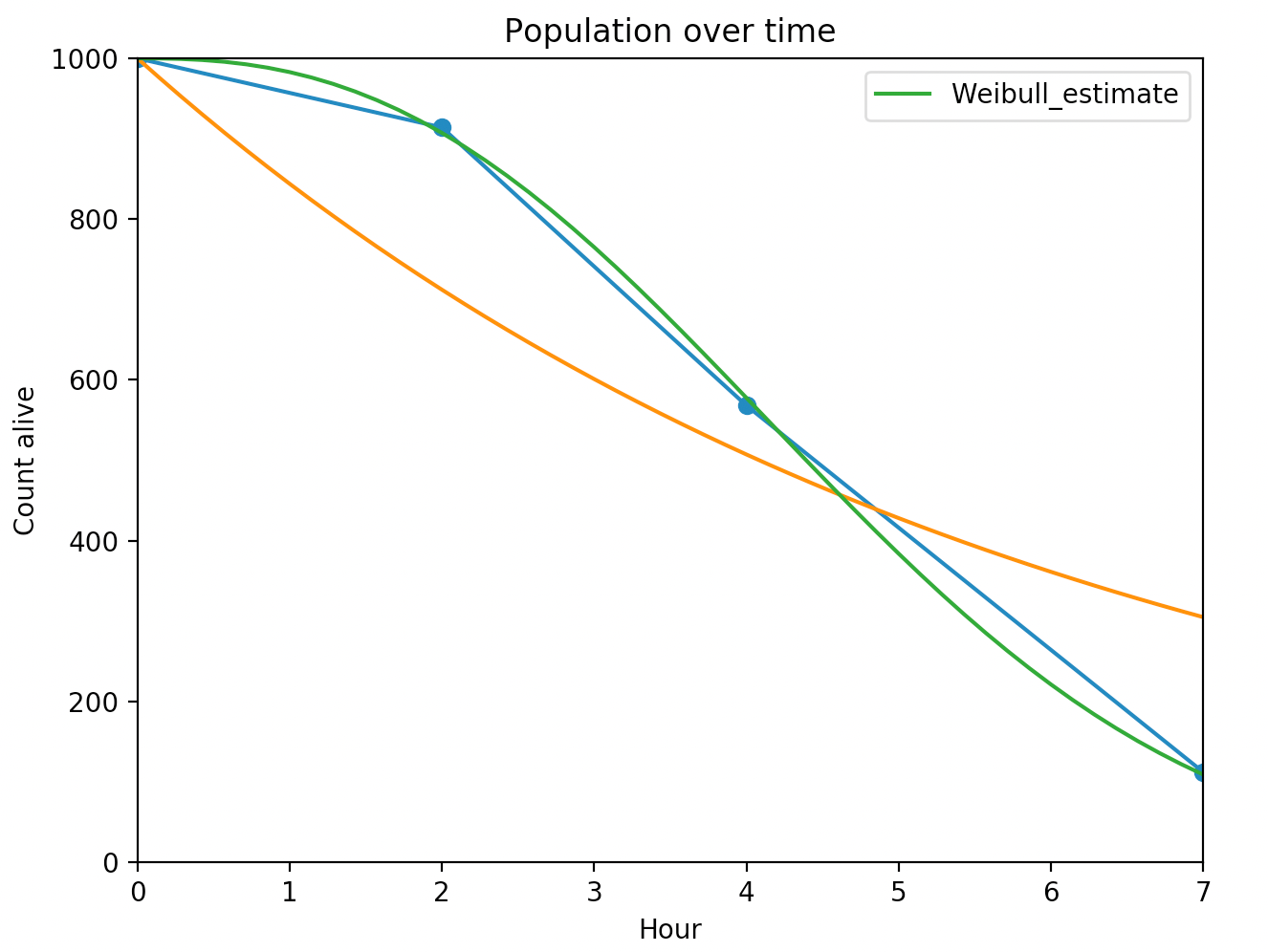Let’s say you have an initial population of (micro-)organisms, and you are curious about their survival rates. A common summary statistic of their survival is the half-life. How might you collect data to measure their survival? Since we are dealing with micro-organisms, we can’t track individual lifetimes. What we might do is periodically count the number of organisms still alive. Suppose our dataset looks like:

T = [0,    2,   4,   7  ]  # in hours
N = [1000, 914, 568, 112]

I’ll present two so-so solutions to finding the half-life, and then one much better solution.

## Solution 1: Linear interpolation

We can plot this over time:We can eyeball the half-life to be about 4.3h - note that this is a linear interpretation between two points, and thus this method doesn’t consider all the data (i.e. it’s not a global method).

## Solution 2: curve-fitting an exponential model

Exponential death is a common model in ecology because of it’s simplicity. We can try to find a $$\beta$$ value such that the sum of squares of observed values minus $$1000 \exp(\beta t)$$ is minimized. That’s not hard to do with scipy’s minimize functions. The best estimate for $$\beta$$ is about 0.17. But, as we can see, it’s not a very good fit:We could choose a more flexible model (like a Weibull distribution), but we will still run up against a fundamental problem: the variance on the estimates will be very high because we are only considering 4 data points, and adding more parameters to the fitting model will only make things worse.

## Solution 3: consider the entire population and censoring

Think again about how the data was collected for a moment. We took a count, waited a few hours, and then took a count again. The delta in the population are composed of individuals that died sometime in that interval. So what we have is a case of interval censoring. That is, I know that 86 individuals died sometime between 0 and 2 (lower and upper bound), 346 died sometime between 2 and 4 (lower and upper bound), etc. Finally, we are left with 112 that are right censored, that is, we stopped watching and don’t observe their death.

We can use survival analysis to answer this problem (hey, let’s use lifelines!). Importantly, we can treat all 1000 organisms as individual observations to make the inference stronger. First, we’ll do some data manipulation.

df = pd.DataFrame({
"deltas": [86, 346, 456, 112],
"start":  [0,  2,   4,   7],
"stop":   [2,  4 ,  7,   np.inf],
"observed_death": [False, False, False, False]
})

print(df)
"""
deltas  start  stop  observed_death
0      86      0   2.0           False
1     346      2   4.0           False
2     456      4   7.0           False
3     112      7   inf           False
"""

We have observed_death as always False, because we don’t have exact measurements on any of the deaths (that is, I have no organisms where I can say “this guy lived exactly X hours”). Why do I have the infinity in the last row? Well, we don’t observe the final 112 organisms die either, but we know they will die between hour 7 and infinity, so we code that in too.

from lifelines import WeibullFitter

wf = WeibullFitter()

wf.fit_interval_censoring(
df['start'],
df['stop'],
df['observed_death'],
weights=df['deltas']
)

wf.print_summary()

"""
<lifelines.WeibullFitter: fitted with 4 observations, 4 censored>
number of subjects = 4
number of events = 0
log-likelihood = -1182.357
hypothesis = lambda_ != 1, rho_ != 1

---
coef  se(coef)  lower 0.95  upper 0.95      p  -log2(p)
lambda_  5.09      0.07        4.94        5.23 <0.005       inf
rho_     2.49      0.08        2.35        2.64 <0.005    282.95
"""

Note the very small standard errors. And if we plot the resulting survival curve, we see an excellent fit.From this, we can report the half-life, or even better is to present the survival distribution, since it has the most information in it.

## Multiple cultures

Let's say we actually have two cultures we are working with, and take population measurements at the same time. However we have different environments for each of the cultures: one has double the sugar concentration in the medium than the other. We can model this using a regression survival model:

 

culture_1 = pd.DataFrame({
"deltas": [86, 346, 456, 112],
"start":  [0,  2,   4,   7],
"stop":   [2,  4 ,  7,   np.inf],
"conc_sugars": [0.15, 0.15, 0.15, 0.15],
"observed_death": [False, False, False, False]
})

culture_2 = pd.DataFrame({
"deltas": [50, 202, 566, 182],
"start":  [0,  2,   4,   7],
"stop":   [2,  4 ,  7,   np.inf],
"conc_sugars": [0.30, 0.30, 0.30, 0.30],
"observed_death": [False, False, False, False]
})
df = pd.concat([culture_2, culture_1])

from lifelines import WeibullAFTFitter

w_aft = WeibullAFTFitter().fit_interval_censoring(df, lower_bound_col='start',                                                       upper_bound_col='stop',                                                       event_col='observed_death',                                                       weights_col='deltas')w_aft.params_"""lambda_ conc_sugars 0.901763         _intercept 1.499147rho_     _intercept 0.996401"""
 

So what is the impact of sugar on microorganisms death? The coefficient associated with sugar concentration in this AFT model is interpreted as "higher means live longer". We get a coefficient of 0.90. Thus organisms with 0.15 sugar concentration "experience" time at a 14.4% faster rate than organisms with 0.30 sugar concentration (Why? $$\exp(0.90 (0.30-0.15))=1.144$$ ).

## Conclusion

This is a simple case of when you want to use interval censoring instead of more naive ways. By thinking about the data generating process, we gain lots of statistical efficiency and better predictions.